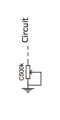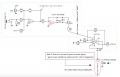# LM13700 Voltage controlled Resistor/Potentiometer with Antilog behaviour

#### jacopo1919

Joined Apr 12, 2020
80
Hello everybody.
I want to replace a C500k variable resistor...with an LM13700 OTA wired as a voltage controlled resistorbased on the single ended VCR of this TI Datasheet

With 0.5mA to 0 current (0.5ma correspond to 10k and as the current decreases, the resistance out of the OTA increases) and given a linear signal, i get a linear resistance response. (from 10k to range of megaohms).

In order to make the external control voltage and VR1 act as their antilog function
(example: given 0-12V coming out of U1.1, if Vin is 1V, Vexponential is 2V or if Vin is 6V, Vexponential is 10V, if Vin is 12V, Vexponential is 12V ),
i believe that i need to convert the mix sum of U1.1 with it's EXPONENTIAL (here an example) curve sticking to the 0-12V range.

Is that correct or i need to translate it to its ANTILOG curve? (using a Log converter circuit)
i'm confused at this point since i would need the values to behave almost exponentiallyish respect to the input but the pot curve is C/antilog.